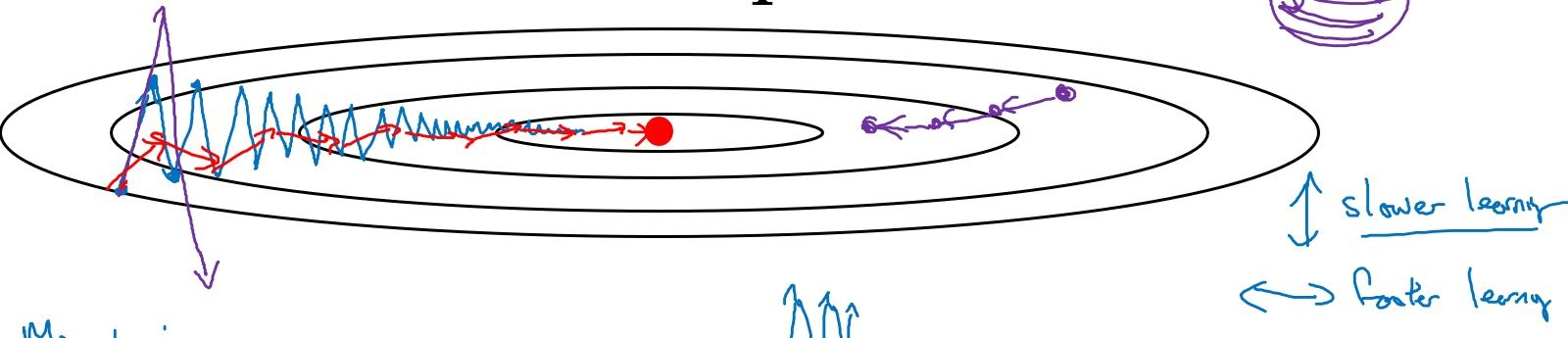Categories:

Updated:

In practice, simple gradient descent (SGD) can face difficulties. Local minimum is rarely a problem because with many features, there hardly is a local minimum where a gradient descent might be trapped in. However, plateau, where gradients are close to 0 is a problem. SGD takes a long time to descend from a plateau.As you can see, there are another optimization algorithms that are faster than SGD.

Here, we will look at Momentum, RMSProp amd Adam.

# Momentum

In SGD, only current $dW$ was considered in one gradient descent step($W:=W-\alpha dW$).

However in Momentum, average of previous $dW$s also have impact in current gradient descent step. We calculate ‘exponentially weighted averages’ of $dW$s and $db$s then use them to update $W$ and $b$.

This is Momentum algorithm;

1. Initialize $v_{dW}$ and $v_{db}$ to 0s.
2. $v_{dW} = \beta_1 v_{dW} + (1-\beta_1)dW$
3. $v_{db} = \beta_1 v_{db} + (1-\beta_1)db$
4. $W = W-\alpha v_{dW}$
5. $b = b-\alpha v_{db}$Look at the contour line of cost with respect to $W$(x-axis) and $b$(y-axis). If we optimize with SGD, our gradient descent will look like blue line, and when we overtune $\alpha$, it might overshoot like purple line on the left. It is reasonable to gradient descent like red line. We are doing this by Momentum. Average of $db$s is close to 0 but average of $dW$s is large. Thus we take big steps in updating $W$, and small steps in updating $b$.

# RMSProp

RMSProp is similar to Momentum but it considers squared average of previous parameters.

This is RMSProp algorithm;

1. Initialize $s_{dW}$ and $s_{db}$ to 0s.
2. $s_{dW} = \beta_2 s_{dW} + (1-\beta_2)dW^2$
3. $s_{db} = \beta_2 s_{db} + (1-\beta_2)db^2$
4. $W = W-\alpha \frac{dW}{\sqrt{s_{dW}+\epsilon}}$
5. $b = b-\alpha \frac{db}{\sqrt{s_{db}+\epsilon}}$

Explanations for step 4 and 5.

• We add $\epsilon$ to the denominator to prevent calculation error from dividing with a value too close to 0.
• In the above image, average of $db^2$ is large, so $s_{db}$ is large and $\frac{db}{\sqrt{s_{db}+\epsilon}}$ is small. Thus $b$ moves a little. It’s the opposite for $W$.

In practice in training neural networks, we use Adam optimizer for default.

Adam optimization algorithm is a combination of Momentum and RMSProp.

1. Initialize $s_{dW}, s_{db}, v_{dW}, v_{db}$ to 0s.
2. $v_{dW}=\beta_1v_W+(1-\beta_1)dW$
3. $v_{dW}^{corrected}=\frac{v_{dW}}{1-{\beta_1}^t}$
4. $s_{dW} = \beta_2 s_{dW} + (1-\beta_2)dW^2$
5. $s_{dW}^{corrected}=\frac{s_{dW}}{1-{\beta_2}^t}$
6. $W:=W-\alpha \frac{v_{dW}^{corrected}}{\sqrt{s_{dW}+\epsilon}}$
7. Do the same with $b$.

We do step 3 and 5 to correct bias of exponentially weighted average.

# Choice of Hyperparameters

This is the typical value of hyperparameters;

$\beta_1=0.9$

$\beta_2=0.999$

$\epsilon =10^{-8}$

Categories: